In :
%load_ext watermark
%watermark -a 'cs224' -u -d -v -p numpy,scipy,pandas,matplotlib,seaborn,pymc3,statsmodels,simdkalman

cs224
last updated: 2020-07-08

CPython 3.6.10
IPython 7.15.0

numpy 1.18.1
scipy 1.4.1
pandas 1.0.4
matplotlib 3.2.1
seaborn 0.10.1
pymc3 3.9.1
statsmodels 0.11.1
simdkalman 1.0.1

In :
%matplotlib inline
import numpy as np, scipy, scipy.stats as stats, scipy.special, scipy.misc, pandas as pd, matplotlib.pyplot as plt, seaborn as sns
import matplotlib as mpl
import sympy

pd.set_option('display.max_columns', 500)
pd.set_option('display.width', 1000)
# pd.set_option('display.float_format', lambda x: '%.2f' % x)
#np.set_printoptions(edgeitems=50)
np.set_printoptions(suppress=True)
np.set_printoptions(edgeitems=30, linewidth=100000) #,  formatter=dict(float=lambda x: "%.3g" % x)
np.core.arrayprint._line_width = 500

sns.set()

from IPython.display import display, HTML

display(HTML("<style>.container { width:70% !important; }</style>"))

In :
import pymc3 as pm
from theano import tensor as tt
import theano

import statsmodels.api as sm
import statsmodels.formula.api as smf

import simdkalman


# Reproducing the "Local level - Nile" State Space Model (Kalman Filter) in PyMC3¶

## The local level model is¶

\begin{align} y_t & = \mu_t + \varepsilon_t, \qquad \varepsilon_t \sim N(0, \sigma_\varepsilon^2) \\ \mu_{t+1} & = \mu_t + \eta_t, \qquad \eta_t \sim N(0, \sigma_\eta^2) \\ \end{align}

## The local level model via statsmodels¶

In :
# This dataset is available in Statsmodels
nile.index = pd.date_range('1871', '1970', freq='AS')

# Plot the series to see what it looks like
fig, ax = plt.subplots(figsize=(32, 8), dpi=80)
ax.plot(nile.index, nile, label='Annual flow volume')
ax.legend()

Out:
<matplotlib.legend.Legend at 0x7ff2c045bc50>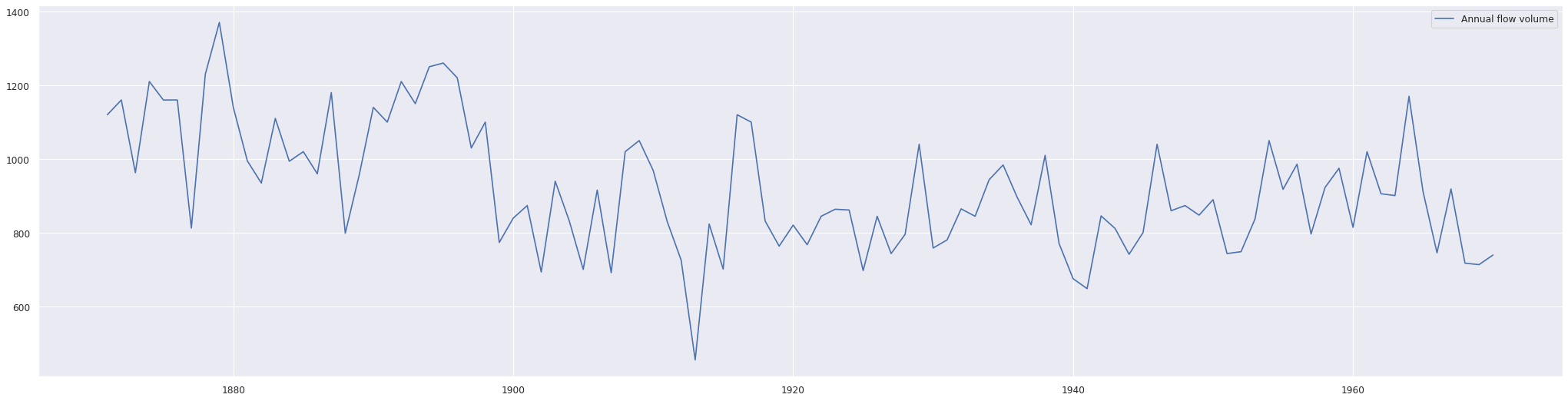In :
# Fit the local level model via the statsmodels implementation
model_ll = sm.tsa.UnobservedComponents(nile, 'local level')
model_fit = model_ll.fit() # (maxiter=200, disp=False)

In :
print(model_fit.summary())

                        Unobserved Components Results
==============================================================================
Dep. Variable:                 volume   No. Observations:                  100
Model:                    local level   Log Likelihood                -632.538
Date:                Wed, 08 Jul 2020   AIC                           1269.076
Time:                        13:04:20   BIC                           1274.266
Sample:                    01-01-1871   HQIC                          1271.176
- 01-01-1970
Covariance Type:                  opg
====================================================================================
coef    std err          z      P>|z|      [0.025      0.975]
------------------------------------------------------------------------------------
sigma2.irregular  1.508e+04   2586.506      5.829      0.000       1e+04    2.01e+04
sigma2.level      1478.8115    851.329      1.737      0.082    -189.762    3147.385
===================================================================================
Ljung-Box (Q):                       35.98   Jarque-Bera (JB):                 0.04
Prob(Q):                              0.65   Prob(JB):                         0.98
Heteroskedasticity (H):               0.61   Skew:                            -0.03
Prob(H) (two-sided):                  0.17   Kurtosis:                         3.08
===================================================================================

Warnings:
 Covariance matrix calculated using the outer product of gradients (complex-step).

In :
model_fit.plot_diagnostics(figsize=(32, 8));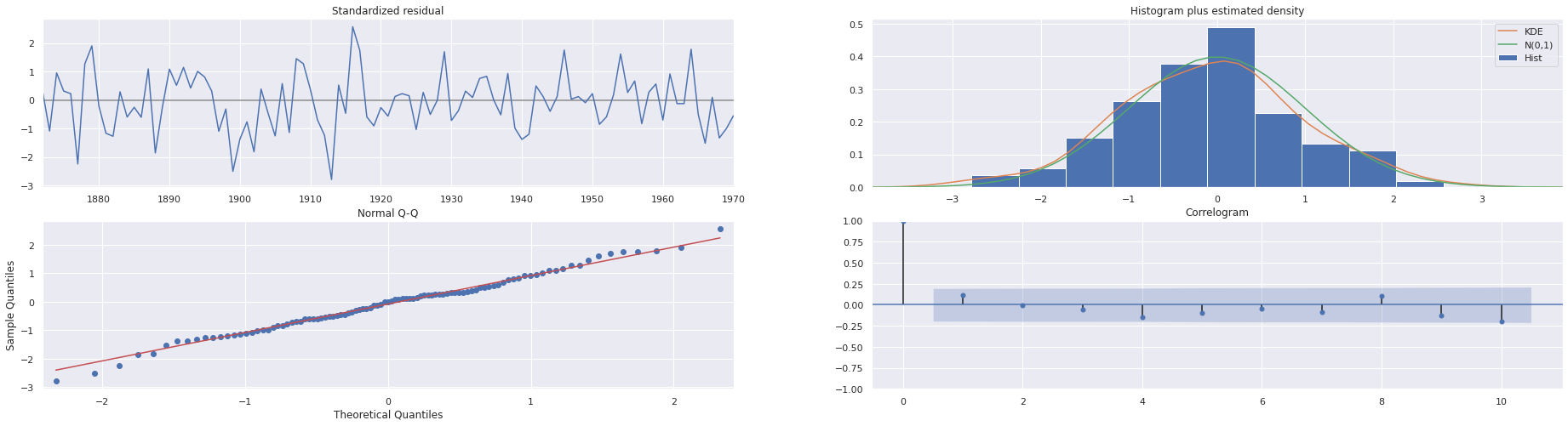In :
fig = model_fit.plot_components(figsize=(32, 8))
fig.tight_layout()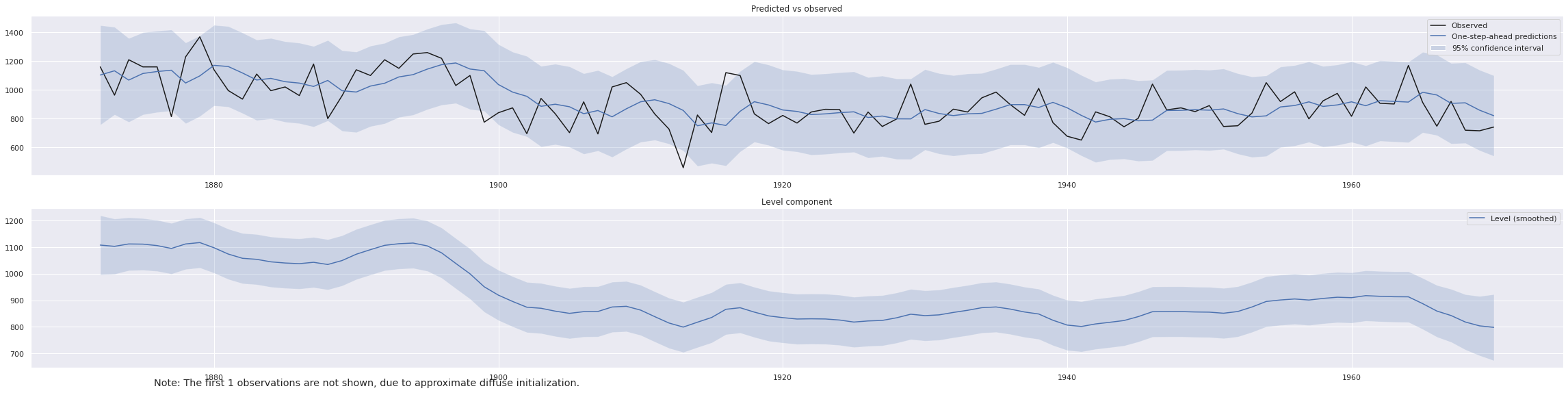In :
ldf = nile.to_frame()
ldf['filtered'] = model_fit.filtered_state
ldf['smoothed'] = model_fit.smoothed_state

In :
fig, ax = plt.subplots(figsize=(32, 8), dpi=80)
ldf.plot(ax=ax)
# ax.legend()

Out:
<matplotlib.axes._subplots.AxesSubplot at 0x7ff2c015cc50>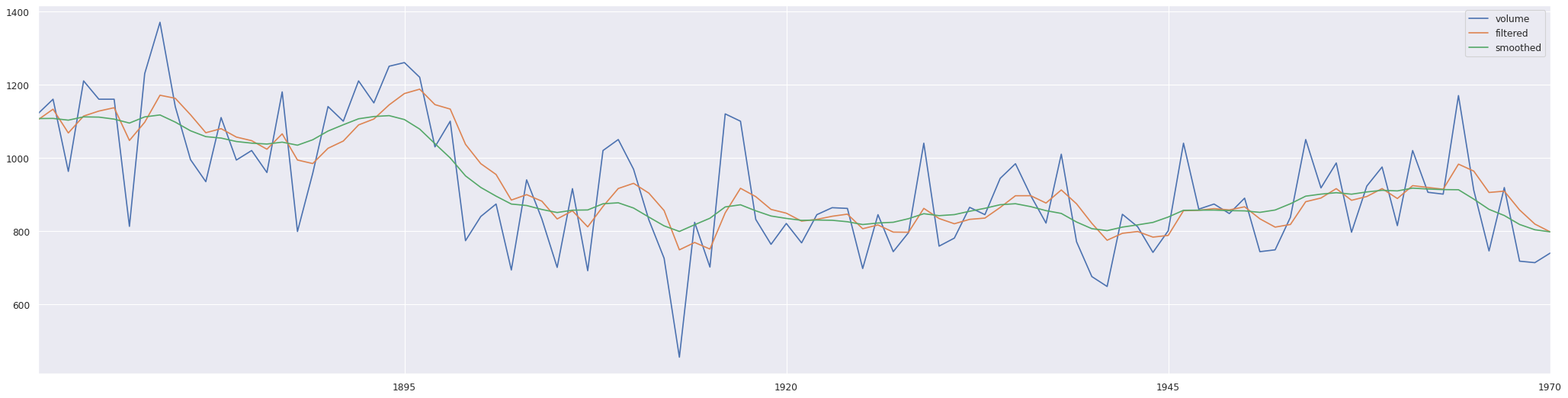## The local level model via PyMC3¶

\begin{align} y_t & = \mu_t + \varepsilon_t, \qquad \varepsilon_t \sim N(0, \sigma_\varepsilon^2) \\ \mu_{t+1} & = \mu_t + \eta_t, \qquad \eta_t \sim N(0, \sigma_\eta^2) \\ \end{align}

Let's first test the theano.scan part outside of PyMC3. This was actually the most difficult part to get this working as the theano.scan function behaves somehow unpredictable and the error messages it produces are not really helpful.

• Theano loops:
• Usage examples of Theano loops in PyMC3:
In :
N=10
η = tt.dvector("η")

μ = tt.dscalar("μ")

def fn_μ_t(η_t, μ_previous):
μ = μ_previous + η_t
return μ

# results, updates = theano.scan(fn_μ_t, sequences = [η], outputs_info = [ dict(initial =  nile.iloc)])
mu, updates = theano.scan(fn_μ_t, sequences = [η], outputs_info = [μ], n_steps=N)

# compute_elementwise(np.array([-1.0]), η0)
η0 = np.arange(N)
μ0 = 0.0
compute_elementwise(μ0, η0)

Out:
[array([ 0.,  1.,  3.,  6., 10., 15., 21., 28., 36., 45.])]

Now let's build the local-level model in PyMC3:

In :
with pm.Model() as m1:
m1_σ_ε = pm.HalfCauchy('σ_ε', beta=1.)
m1_σ_η = pm.HalfCauchy('σ_η', beta=1.)
m1_η = pm.Normal('η', mu=0, sigma=m1_σ_η, shape=len(nile))
m1_μ = theano.shared(nile.iloc)

m1_mu, m1_updates = theano.scan(fn=fn_μ_t, sequences = [m1_η], outputs_info = [m1_μ], n_steps=len(nile))
m1_mu_ = pm.Deterministic('μ', m1_mu)
m1_level = pm.Normal('ll', mu=m1_mu_, sigma=m1_σ_ε, observed=nile)


And finally run the sampling process:

In :
with m1:
m1_trace = pm.sample()

Auto-assigning NUTS sampler...
Initializing NUTS using jitter+adapt_diag...
Multiprocess sampling (4 chains in 4 jobs)
NUTS: [η, σ_η, σ_ε]

100.00% [8000/8000 01:37<00:00 Sampling 4 chains, 202 divergences]
Sampling 4 chains for 1_000 tune and 1_000 draw iterations (4_000 + 4_000 draws total) took 98 seconds.
There were 8 divergences after tuning. Increase target_accept or reparameterize.
There were 16 divergences after tuning. Increase target_accept or reparameterize.
There were 178 divergences after tuning. Increase target_accept or reparameterize.
The acceptance probability does not match the target. It is 0.8965076879587853, but should be close to 0.8. Try to increase the number of tuning steps.
The rhat statistic is larger than 1.05 for some parameters. This indicates slight problems during sampling.
The estimated number of effective samples is smaller than 200 for some parameters.

In :
pm.summary(m1_trace, var_names=['σ_ε', 'σ_η'])

Out:
mean sd hdi_3% hdi_97% mcse_mean mcse_sd ess_mean ess_sd ess_bulk ess_tail r_hat
σ_ε 124.559 12.670 97.861 146.472 1.517 1.077 70.0 70.0 75.0 32.0 1.05
σ_η 32.838 13.598 9.333 55.719 1.947 1.385 49.0 49.0 50.0 196.0 1.08

Let's compare the PyMC3 values for $\sigma_\varepsilon$ and $\sigma_\eta$ to the statsmodels values. We need to take the square root as statsmodels is providing variances (indicated by the name sigma2):

In :
np.sqrt(model_fit.params)

Out:
sigma2.irregular    122.792551
sigma2.level         38.455318
dtype: float64

The match is quite good.

In :
m1_trace['μ'].shape

Out:
(4000, 100)
In :
ldf['pymc3'] = m1_trace['μ'].mean(axis=0)

In :
fig, ax = plt.subplots(figsize=(32, 8), dpi=80)
ldf[['volume', 'filtered', 'smoothed', 'pymc3']].plot(ax=ax)

Out:
<matplotlib.axes._subplots.AxesSubplot at 0x7ff2a78320f0>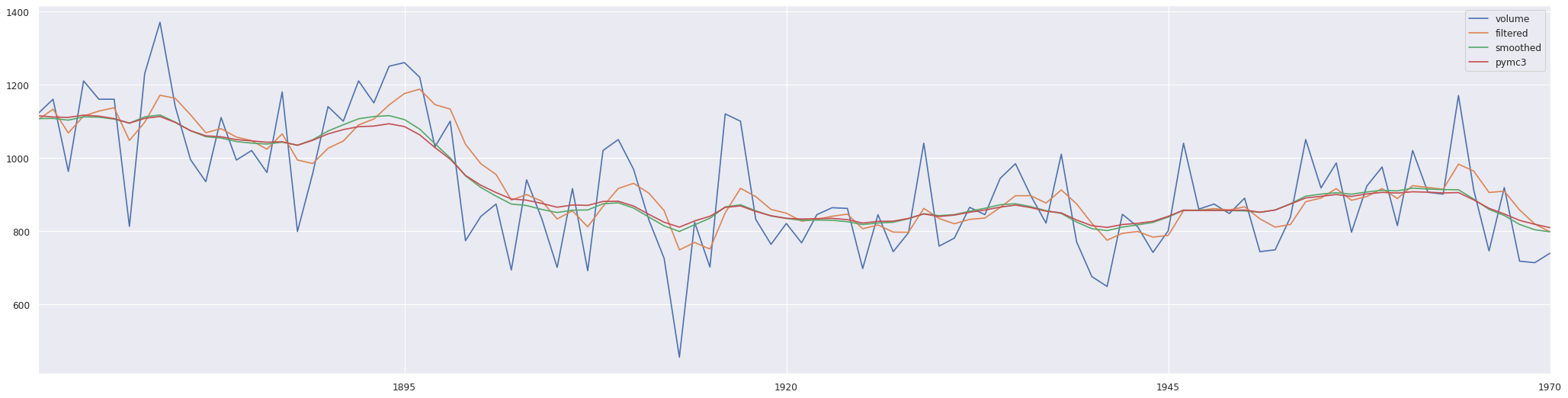As you can see the PyMC3 implementation matches ver much the result of the statsmodels smoothing implementation. Statsmodels is of course much faster, but PyMC3 is more flexible, e.g. in case that you do not have normally distributed error terms but let's say heavy tailed Student's t-distributed error terms the adaptation of the PyMC3 model is a child's play.

### Why do more tune steps lead to a higher r_hat?¶

In :
with m1:
m1_trace_ = pm.sample(1000, tune=3000)

Auto-assigning NUTS sampler...
Initializing NUTS using jitter+adapt_diag...
Multiprocess sampling (4 chains in 4 jobs)
NUTS: [η, σ_η, σ_ε]

100.00% [16000/16000 03:06<00:00 Sampling 4 chains, 107 divergences]
Sampling 4 chains for 3_000 tune and 1_000 draw iterations (12_000 + 4_000 draws total) took 188 seconds.
There were 44 divergences after tuning. Increase target_accept or reparameterize.
There were 5 divergences after tuning. Increase target_accept or reparameterize.
The acceptance probability does not match the target. It is 0.9069628598757123, but should be close to 0.8. Try to increase the number of tuning steps.
There were 5 divergences after tuning. Increase target_accept or reparameterize.
There were 53 divergences after tuning. Increase target_accept or reparameterize.
The rhat statistic is larger than 1.05 for some parameters. This indicates slight problems during sampling.
The estimated number of effective samples is smaller than 200 for some parameters.

In :
pm.summary(m1_trace_, var_names=['σ_ε', 'σ_η'])

Out:
mean sd hdi_3% hdi_97% mcse_mean mcse_sd ess_mean ess_sd ess_bulk ess_tail r_hat
σ_ε 125.06 12.003 104.197 148.595 0.920 0.652 170.0 170.0 171.0 386.0 1.02
σ_η 34.11 14.309 11.608 58.505 1.901 1.351 57.0 57.0 48.0 131.0 1.07

## The local level model via Kalman Filter¶

This section is just to show that the statsmodels implementation is a Kalman filter. A raw Kalman filter cannot perform the kind of "higher-order" parameter fitting that the statsmodels implementation does. Therefore we just copy the fitted parameters over from the statsmodels fit.

• simdkalman
In :
lds = nile

nile_kf = simdkalman.KalmanFilter(
state_transition=np.array([]),
observation_model=np.array([]),
process_noise=np.diag([1478.8115]),
observation_noise=1.508e+04
)
# fit noise parameters to data with the EM algorithm (optional)
# nile_kf = nile_kf.em(lds, n_iter=10)
nile_smoothed = nile_kf.smooth(lds, initial_value=nile.iloc, initial_covariance=1.0)

ldf['kf'] = nile_smoothed.observations.mean

In :
fig, ax = plt.subplots(figsize=(32, 8), dpi=80)
ldf[['volume', 'smoothed', 'kf']].plot(ax=ax)

Out:
<matplotlib.axes._subplots.AxesSubplot at 0x7ff2ac19c128>